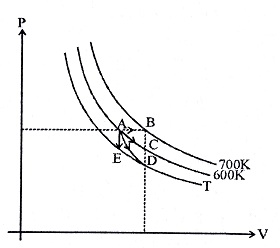${H}_{2}\left(g\right)+\frac{1}{2}{O}_{2}\left(g\right)\to {H}_{2}O\left(l\right)$

B.E. (H-H) = x1; B.E. (O=O) = x2 B.E. (O-H) = x3

Latent heat of vaporization of water liquid into water vapour = x4, then $∆{H}_{f}$(heat of formation of liquid water) is-

1. ${x}_{1}+\frac{{x}_{2}}{2}-{x}_{3}+{x}_{4}$

2. $2{x}_{3}-{x}_{1}-\frac{{x}_{2}}{2}-{x}_{4}$

3. ${x}_{1}+\frac{{x}_{2}}{2}-2{x}_{3}-{x}_{4}$

4. ${x}_{1}+\frac{{x}_{2}}{2}-2{x}_{3}+{x}_{4}$

Concept Questions :-

Enthalpy and It's Type
High Yielding Test Series + Question Bank - NEET 2020

Difficulty Level:

In a process the pressure of a gas is inversely proportional to the square of the volume. If temperature of the gas is increases, then work done on the gas-

1. is positive

2. is negative

3. is zero

4. may be positive

Concept Questions :-

Thermodynamics' Properties and process
High Yielding Test Series + Question Bank - NEET 2020

Difficulty Level:

The enthalpies of formation of CO2(g) and CO(g) at 298 K are in the ratio 2.57 : 1. For the reaction,

1. -150.6 kJ mol-1

2. -109.8 kJ mol-1

3. -130.2 kJ mol-1

4. -141.8 kJ mol-1

High Yielding Test Series + Question Bank - NEET 2020

Difficulty Level:

In an adiabatic expansion the product of pressure and volume-

1. decreases

2. increases

3. remains constant

4. first increase then decreases

High Yielding Test Series + Question Bank - NEET 2020

Difficulty Level:

The molar entropy of vapourisation of acetic acid is 144.4 cal K-1 mol-1 at its boiling point ${118}^{\circ }C$. The latent heat of vapourisation of acetic acid is

1. 49 cal g-1

2. 64 cal g-1

3. 94 cal g-1

4. 84 cal g-1

High Yielding Test Series + Question Bank - NEET 2020

Difficulty Level:

100 ml of 0.3 M HCl solution is mixed with 100 ml of 0.35 M NaOH solution. The amount of heat liberated is

1. 7.3 kJ

2. 5.71 kJ

3. 10.42 kJ

4. 1.713 kJ

High Yielding Test Series + Question Bank - NEET 2020

Difficulty Level:

Five moles of ideal gas expand isothermally and reversibly from an initial pressure of 100 atm to a final pressure of 1 atm at ${27}^{\circ }C$. The work done by the gas is

1. 3455 cal

2. 6909 cal

3. 0

4. 69010 cal

High Yielding Test Series + Question Bank - NEET 2020

Difficulty Level:

The quantity ${\delta }_{q}$ i.e. heat absorbed an infinitesimal process is

1. Dependent on the path of transformation

2. Dependent on the thermodynamic state of the system

3. Independent of both

4. An exact differential

High Yielding Test Series + Question Bank - NEET 2020

Difficulty Level:

An ideal gas absorbs 2000 cals of heat from a heat reservoir and does mechanical work equivalent 4200 J. The change in internal energy of the gas is

1. 3000 cals

2. 2000 cals

3. 1500 cals

4. 1000 cals

High Yielding Test Series + Question Bank - NEET 2020

Difficulty Level:

For an ideal gas four processes are marked as 1, 2, 3 and 4 on P-V diagram as shown in figure. The amount of heat supplied to the gas in the process 1, 2, 3 and 4 are Q1, Q2, Q3 and Q4 repectively, then correct order of heat supplied to the gas is

[AB is process-1, AC is process-2, AD is adiabatic process-3 and AE is process-4]1. ${Q}_{1}>{Q}_{2}>{Q}_{3}>{Q}_{4}$

2. ${Q}_{1}>{Q}_{2}>{Q}_{4}>{Q}_{3}$

3.  ${Q}_{1}>{Q}_{4}>{Q}_{2}>{Q}_{4}$

4. ${Q}_{1}<{Q}_{2}<{Q}_{3}<{Q}_{4}$